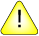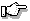This version of this document is no longer maintained. For the latest documentation, see http://www.qnx.com/developers/docs.

sinh(), sinhf()

Compute the hyperbolic sine

Synopsis:

#include <math.h>

double sinh( double x );

float sinhf( float x );

Arguments:

x
The angle, in radians, for which you want to compute the hyperbolic sine.

Library:

libm

Use the -l m option to qcc to link against this library.

Description:

The sinh() and sinhf() functions compute the hyperbolic sine (specified in radians) of x. A range error occurs if the magnitude of x is too large.

Returns:

The hyperbolic sine value.If an error occurs, these functions return 0, but this is also a valid mathematical result. If you want to check for errors, set errno to 0, call the function, and then check errno again. These functions don't change errno if no errors occurred.

Examples:

#include <stdio.h>
#include <math.h>
#include <stdlib.h>

int main( void )
{
printf( "%f\n", sinh(.5) );

return( EXIT_SUCCESS );
}

produces the output:

0.521095

Classification:

Safety:
Cancellation point No
Interrupt handler No
Signal handler No